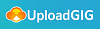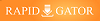# Udemy - Learn Basics of Calculus

#### Category: Tutorial

Posted on 2021-10-25, by voska89.

DescriptionMP4 | Video: h264, 1280x720 | Audio: AAC, 44.1 KHz
Language: English | Size: 1.50 GB | Duration: 8h 54m
Introduction to Limits and Derivatives

What you'll learn
In this course the students will learn about Introduction to limits and their applications along with Introduction to Derivatives with applications.
Objective of this course is to teach in a simple and easiest way so that every student can understand and pickup the concepts even if he has never studied mathe
The questions are practically demonstrated how they are solved.
By solving the questions the students how have fear that calculus is very difficult is removed, and they can solve simple questions by themselves.
After learning the students will be able practically apply and analyze in their Daily Job.
Requirements
Basics Knowledge of Arithmatics, like addition, subtraction etc. and some basic algebraic formulas.
Description
This course has been designed keeping in view the difficulties faced by students in understanding and solving the questions. This course has been outlined for the students of A-level, BS, BBA, Engineering, rather any field where the concepts of calculus are applied. Also those who never studied calculus or consider it very difficult to understand will feel comfortable.
Two major topics i.e. LIMITS and DERIVATIVES have been selected with basic questions and techniques to understand concepts and handle problems. after studying these you will be able to apply in your specific field like business, Engineering etc.
The course has been divided into three sections in which:
Section one contains free sample lectures for the students to decide about the course selection.
Section two contains four video lectures on Basics of limits with sufficient number of solved questions, where every step clearly discussed. This section covers:
Basics of Limits. Using Formulas
Sample Questions for solving limits
Limits Involving Infinity
Limits for trigonometric functions
Section three contains ten video lectures on the introduction of derivatives with solved examples.
Introduction to Average Rate of Change.
Instantaneous Rate of Change
Introduction to derivatives Using formulas
Derivatives of Trigonometric Function
Chain Rule
Implicit Differentiation
Derivatives of Natural Logarithms
Derivatives of Exponential Functions
L'Hopitals Rule for evaluating Limits When Undetermined form occurs
Analysis of Function (First order and second order tests have been included.)
Hopefully you will like and consider my course for yourself and for your friends.
Dr. Humayun
Who this course is for:
This course has been desigined for the students who are studying calculus for the first time, and also for those who think it is very difficult to learn.

Homepage
`https://www.udemy.com/course/learn-basics-of-calculus/`More Course Expensive Download Click Here : https://www.ebookee.com/user/voska89

https://hot4share.com/4b2tr9m5fdar/19yr6.Learn.Basics.of.Calculus.part1.rar.html
https://hot4share.com/lcs7a1bm83iu/19yr6.Learn.Basics.of.Calculus.part2.rar.htmlhttps://rapidgator.net/file/1d780e9aec4f41e14ea9cae8f4540e73/19yr6.Learn.Basics.of.Calculus.part1.rar.html
https://rapidgator.net/file/1d00d0c686f23019b082dd8268278e9c/19yr6.Learn.Basics.of.Calculus.part2.rar.html

9406 dl's @ 2043 KB/s
6342 dl's @ 2839 KB/s
8038 dl's @ 3686 KB/s

Search More...
Udemy - Learn Basics of CalculusRelated Books